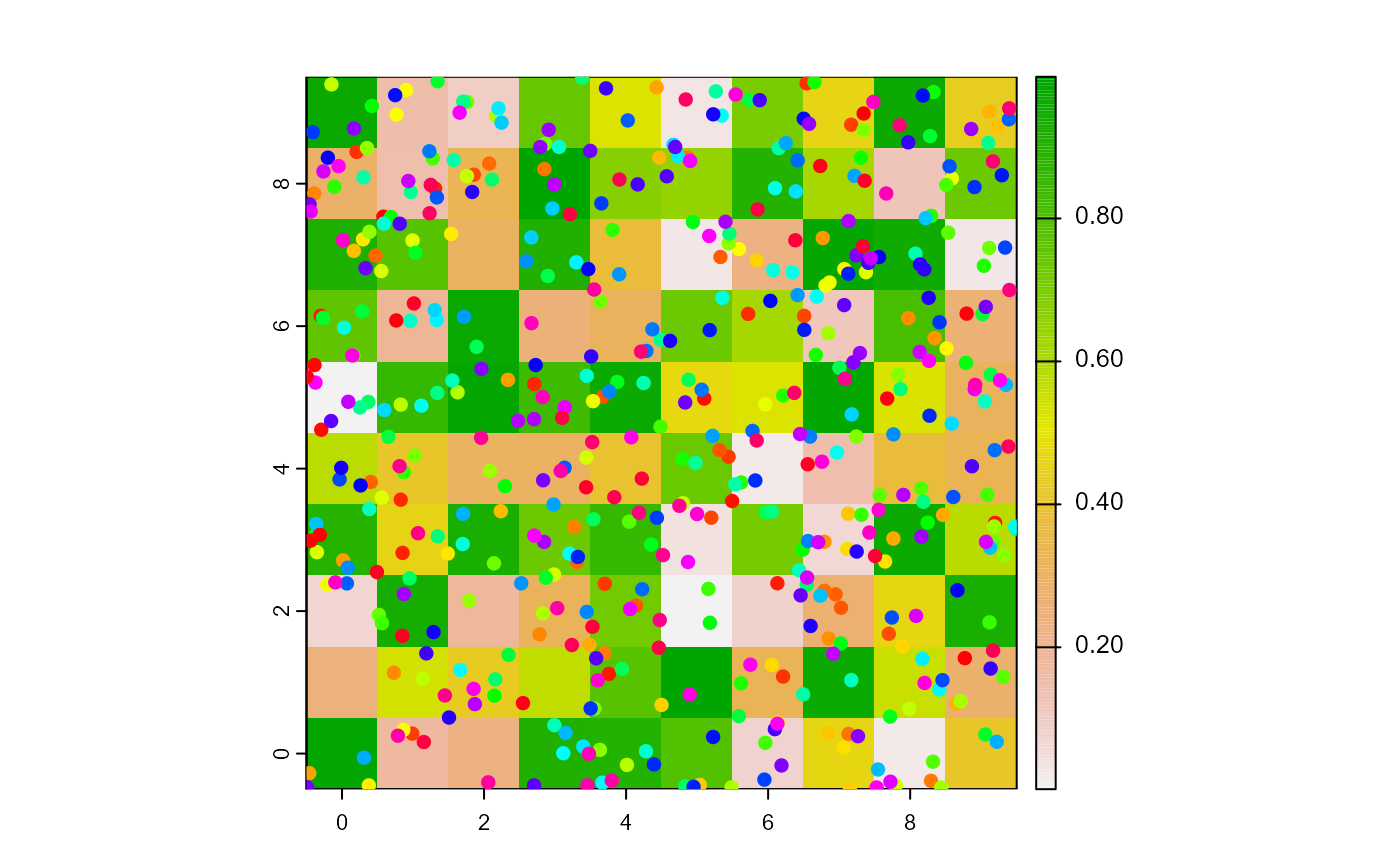Report the individuals among turtles that are on the same patches as the agents.

turtlesOn(world, turtles, agents, breed, simplify = TRUE)

# S4 method for worldNLR,agentMatrix,matrix,missing
turtlesOn(world, turtles, agents, simplify)

# S4 method for worldNLR,agentMatrix,matrix,character
turtlesOn(world, turtles, agents, breed, simplify = TRUE)

## Arguments

world

WorldMatrix or worldArray object.

turtles

AgentMatrix object representing the moving agents.

agents

Matrix (ncol = 2) with the first column pxcor and the second column pycor representing the patches coordinates, or

AgentMatrix object representing the moving agents.

breed

Characters. Vector of breed names for the selected turtles. If missing, there is no distinction based upon breed.

simplify

Logical. If simplify = TRUE, all turtles on the same patches as any agents are returned; if simplify = FALSE, the turtles are evaluated for each agents's patches individually.

## Value

AgentMatrix representing any individuals from turtles of any of the given breed, if specified, located on the same patches as any of the agents, if simplify = TRUE, or Matrix (ncol = 2) with the first column whoTurtles and the second column id showing which turtles are on the same patches as which agents represented by id, if simplify = FALSE. id represents and follows the order of the agents. id does not represent the who numbers of the agents if agents are turtles.

## Details

The agents must be located inside the world's extent.

Sarah Bauduin

## Examples

w1 <- createWorld(minPxcor = 0, maxPxcor = 9, minPycor = 0, maxPycor = 9,
data = runif(100))
t1 <- createTurtles(n = 500, coords = randomXYcor(w1, n = 500))
plot(w1)
points(t1, col = of(agents = t1, var = "color"), pch = 16)t2 <- turtlesOn(world = w1, turtles = t1, agents = patch(w1, 2, 2))